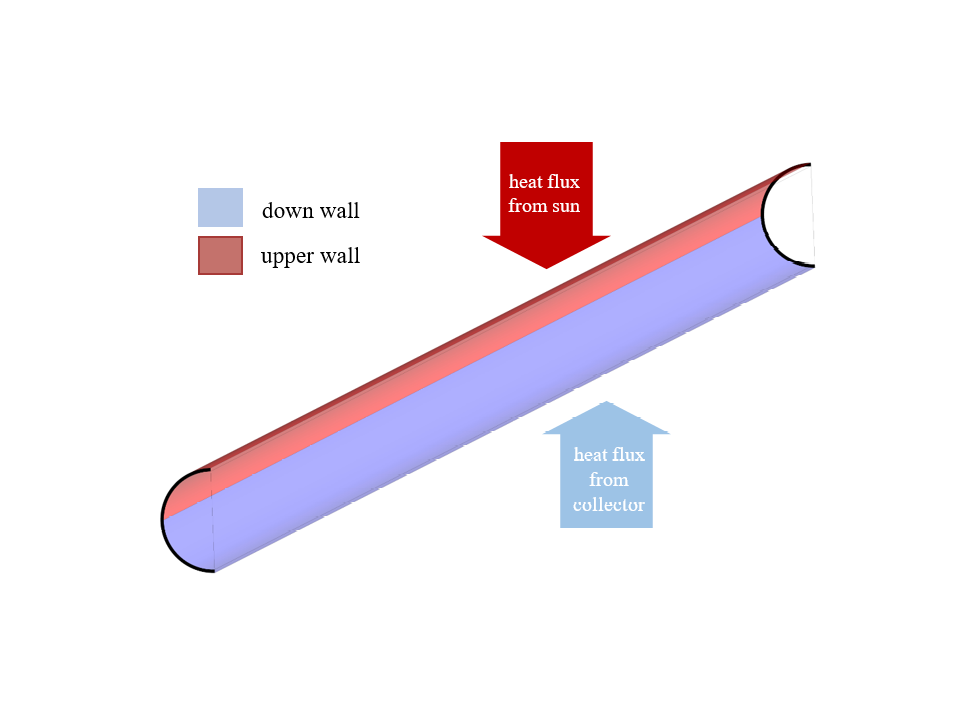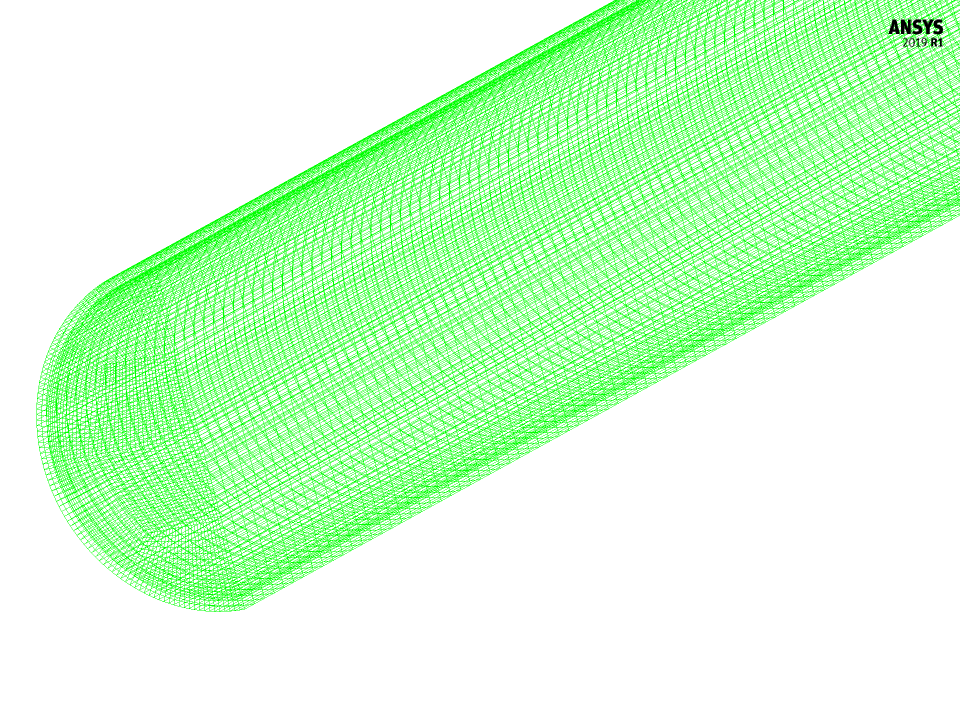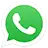# Parabolic Solar Collector with Nano Fluid, Paper Numerical Validation, ANSYS Fluent Training

241.00 \$

The paper “Thermal performance analysis of solar parabolic trough collector using nanofluid as working fluid: A CFD modelling study“, has been simulated and the paper results are validated.

This product includes Geometry & Mesh file and a comprehensive Training Movie.

There are some free products to check the service quality.

To order your ANSYS Fluent project (CFD simulation and training), contact our experts via [email protected], online support, and WhatsApp.

Click on Add To Cart and obtain the Geometry file, Mesh file, and a Comprehensive Training Video.

To Order Your Project or benefit from a CFD consultation, contact our experts via email ([email protected]), online support tab, or WhatsApp at +1 (903) 231-3943.

## Paper Description

The present problem simulates heat transfer within a tube of a parabolic solar collector containing water flow. This numerical simulation has been done based on the reference paper “Thermal performance analysis of solar parabolic trough collector using nanofluid as working fluid: A CFD modelling study“, and the results have been compared and validated with the results in the article by ANSYS Fluent software. In fact, in the current model, there is a tube with a water flow that is exposed to solar energy.

Behind the tube, there is a parabolic plate that absorbs the solar radiant energy, which is responsible for absorbing the heat energy from the sun’s radiation and then reflecting it. In this case, only the water-flowing pipe is modeled; Thus, the wall of the water pipe is divided into two parts, the upper and lower wall. The upper part of the wall is directly exposed to solar energy and receives heat in the form of heat flux from the sun’s rays; While the lower part of the wall is affected by the reflection energy of the parabolic absorber plates of the collector and receives the energy as a constant heat flux.

## Paper Description

Also, the wall of the water pipe is made of aluminum. The inlet water flow to the pipe has a Reynolds of 30,000 and a temperature of 320 K, which according to the relationship between the Reynolds number and the amount of thermophysical properties of the water fluid, the value of the water flow inlet velocity is equal to 0.5024043 m.s-1. Based on the relationships in the paper, it is assumed that the pipe wall has a constant heat flux in both its upper and lower parts; So that for the upper wall, the heat flux is equal to 750 W.m-2 and for the lower wall, the heat flux is equal to 19500 W.m-2. The main purpose of this simulation is to investigate the Nusselt number.

## Parabolic Solar Collector Geometry & Mesh

The present model is designed in three dimensions using Design Modeler software. The geometry of the model consists of a tube which, due to its symmetrical structure, is drawn in a semi-cylindrical shape. The tube consists of two parts, the thin outer layer of which acts as the solid wall of the tube and the inner part which acts as the fluid conduit. The pipe has an internal diameter of 0.06 m and a length of 2 m, which has a thickness of 0.002 m. The following figure shows a view of the geometry.The meshing of the model has been done using ANSYS Meshing software and the mesh type is structured. The element number is 1475000. The following figure shows the mesh.## Parabolic Solar Collector CFD Simulation

To simulate the present model, several assumptions are considered:

• We perform a pressure-based solver.
• The gravity effect on the fluid is ignored.

A summary of the defining steps of the problem and its solution is given in the following table:

 Models Viscous model k-epsilon k-epsilon model RNG near-wall treatment standard wall function Boundary conditions Inlet Velocity inlet velocity magnitude 0.5024043 m.s-1 temperature 320 K Outlet Pressure outlet gauge pressure 0 Pascal Upper Wall Wall wall motion stationary wall heat flux 750 W.m-2 Down Wall wall motion stationary wall heat flux 19500 W.m-2 Solution Methods Pressure-velocity coupling SIMPLE Spatial discretization pressure standard momentum first order upwind energy first order upwind turbulent kinetic energy first order upwind turbulent dissipation rate first order upwind Initialization Initialization method Standard gauge pressure 101325 pascal temperature 320 K z-velocity 0.5024043 m.s-1 x-velocity, y-velocity 0 m.s-1

## Paper Validation

At the end of the solution process, the value of the Nusselt number in the model is calculated and compared and validated with the Nusselt values in the reference article. The amount of surface Nusselt at the level corresponding to the contact boundary between the fluid and the pipe wall is calculated using the REPORT command. Since, according to the paper, the value of the Nusselt number is obtained in the area of fully developed flow, the present numerical simulation also focuses on the value of the Nusselt number at the end of the pipe where the flow is developed.

The table below shows the amount of Nusselt number on the contact surface between water and pipe, which is obtained in different sections of the pipe and at different distances from the outlet section of the pipe. Then the value of the Nusselt number of the article is obtained according to the diagram of Figure 4 of the article and in the value of Reynolds equal to 30,000 of this figure.

## Paper Validation

Comparing the amount of surface Nusselt at different sections at the ending areas of the pipe with the amount of Nusselt in the article indicates that the closer we get to the end of the pipe and the area with the developed flow, the accuracy of the solution and the validation of the present simulation becomes more valid.

 Present Simulation distance to outlet Nusselt Paper Work Nusselt 0 m to outlet 220.0619 221.3 0.05 m to outlet 221.5318 0.1 m to outlet 222.7393 0.15 m to outlet 224.2536 0.2 m to outlet 225.5326 0.25 m to outlet 226.9974 0.3 m to outlet 228.3515 0.35 m to outlet 229.7931 0.4 m to outlet 231.0474 0.45 m to outlet 232.4048 0.5 m to outlet 233.7537

Two-dimensional and three-dimensional contours related to pressure, velocity, and temperature are also obtained. Two-dimensional contours are drawn in the symmetrical cross section of the model.

You can obtain Geometry & Mesh file and a comprehensive Training Movie that presents how to solve the problem and extract all desired results.

## Reviews

There are no reviews yet.Call On WhatsApp